## 《电子技术基础（1）（下）1346》在线作业1

《电子技术基础（1）（下）1346》在线作业1

------------------------
1.集成4位二进制数据比较器为最低位芯片时 ，级联输入端（扩展端）的接法正确的是（ ）。
A.(a>b)=0, (a=b)=0, (a<b)=0
B.(a>b)=1, (a=b)=0, (a<b)=0
C.(a>b)=0, (a=b)=1, (a<b)=0
D.(a>b)=?, (a=b)=?, (a<b)=?

2.图示各逻辑电路中，为一位二进制计数器的是 ( ) 。
A.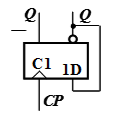B.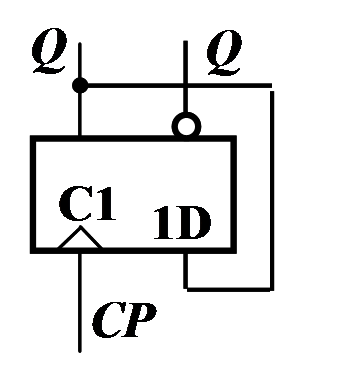C.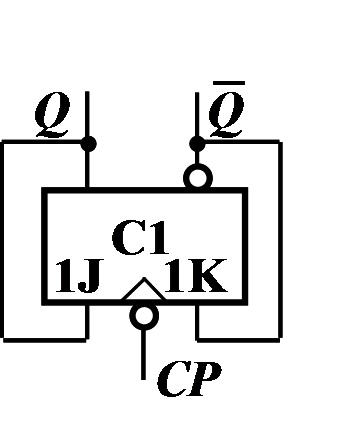D.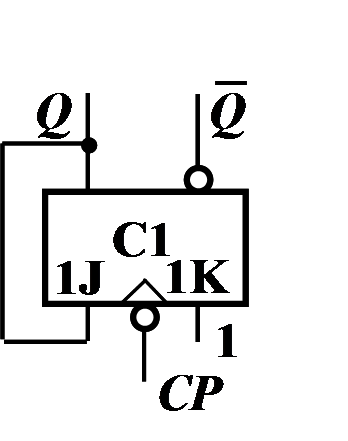3.在下列函数中，F恒为0的是
A.B.C.D.4.A/D转换过程四个步骤的顺序是
A.编码、量化、保持、采样
B.编码、量化、保持、采样
C.采样、编码、量化、保持
D.采样、保持、量化、编码

5.用译码器74138和适当的逻辑门实现函数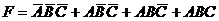，正确的连线电路为（ ）。
A.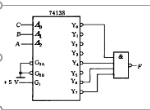B.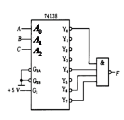C.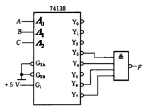D.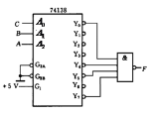6.将矩形波变换三角波，需用
A.RC微分电路
B.RC耦合电路
C.RC积分电路
D.以上都不对

7.D/A转换器的转换精度通常以
A.分辨率形式给出
B.线性度形式给出
C.最大静态转换误差的形式给出
D.二进制数字给出

8.D/A转换器的位数越多，转换精度越（ ）。
A.差
B.高
C.没得影响
D.不确定

9.若译码/驱动器输出为低电平时，显示器发光则显示器应选用
A.共阴极显示器
B.共阳极显示器
C.共阳共阴极显示器都可以
D.不能确定

10.A.图（a）
B.图（a）图（b）都正确
C.图（b）
D.图（a）图（b）都错误

11.欲使一路数据分配到多路装置应选用
A.编码器
B.选择器
C.分配器
D.加法器

12.对于J-K触发器，当输入J=K=1时在时钟脉冲作用下其逻辑功能（ ）。
A.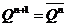B.置0
C.置1
D.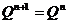13.在下图CMOS电路中实现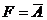电路为（ ）。
A.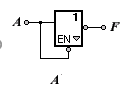B.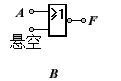C.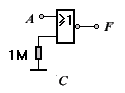D.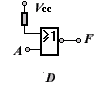14.某同步时序逻辑电路的状态转换图如所示，该时序逻辑电路是（ ）。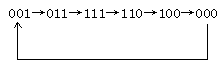A.同步四进制计数器
B.同步六进制计数器
C.同步八进制计数器
D.同步五进制计数器

15.电路如下图所示，当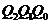的初态为011，在时钟CP脉冲信号作用下，的下一个状态为（ ）。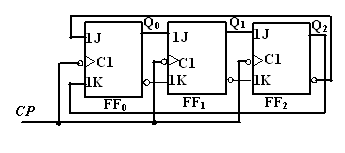A.100
B.101
C.110
D.111

------------------------
1.具有记忆功能的各类触发器是构成时序电路的基本单元。
A.错误
B.正确

2.OC与非门的输出端可以并联运行，实现“线与”关系，即L=L1﹒L2。
T.对
F.错

3.7. 具有移位功能的寄存器，称为移位寄存器。
A.错误
B.正确

4.5. 带使能端的译码器不能作数据分配器使用。
A.错误
B.正确

5.单稳态触发器的暂稳态时间与输入触发脉冲宽度成正比。
T.对
F.错

6.JK触发器只要J，K端同时为1，则一定引起状态翻转。
T.对
F.错

7.13. 多谐振荡器常作为脉冲信号源使用。
A.错误
B.正确

8.方波的占空比为50%。
T.对
F.错

9.D触发器的特性方程为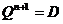，与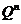无关，所以它没有记忆功能。
T.对
F.错

10.T触发器的特性方程为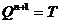T.对
F.错